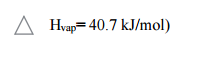# Problem: Calculate the mass of water that can be vaporized with 155 kJ of heat at 100 °C? (Molar Mass of Water= 18.02 g/mol, ΔHvap = 40.7 kJ/mol) a. 12.5 gb. 36.0 gc. 68.6 gd. 86.8 ge. 18.02 g

86% (86 ratings)
###### Problem Details

Calculate the mass of water that can be vaporized with 155 kJ of heat at 100 °C? (Molar Mass of Water= 18.02 g/mol, ΔHvap = 40.7 kJ/mol)

a. 12.5 g

b. 36.0 g

c. 68.6 g

d. 86.8 g

e. 18.02 gWhat scientific concept do you need to know in order to solve this problem?

Our tutors have indicated that to solve this problem you will need to apply the Thermochemical Equation concept. You can view video lessons to learn Thermochemical Equation. Or if you need more Thermochemical Equation practice, you can also practice Thermochemical Equation practice problems.

What is the difficulty of this problem?

Our tutors rated the difficulty ofCalculate the mass of water that can be vaporized with 155 k...as medium difficulty.

How long does this problem take to solve?

Our expert Chemistry tutor, Sabrina took 4 minutes and 1 second to solve this problem. You can follow their steps in the video explanation above.

What professor is this problem relevant for?

Based on our data, we think this problem is relevant for Professor Alghoul's class at GSU.